# Test: Basic FET Amplifiers- 2

## 10 Questions MCQ Test Topicwise Question Bank for Electrical Engineering | Test: Basic FET Amplifiers- 2

Description
Attempt Test: Basic FET Amplifiers- 2 | 10 questions in 30 minutes | Mock test for Electrical Engineering (EE) preparation | Free important questions MCQ to study Topicwise Question Bank for Electrical Engineering for Electrical Engineering (EE) Exam | Download free PDF with solutions
QUESTION: 1

### In the common drain amplifier shown below, if RS = 4 KΩ, RG = 10 MΩ, μ = 50 and rd = 35 KΩ then the voltage gain Av of the amplifier is approximately equal to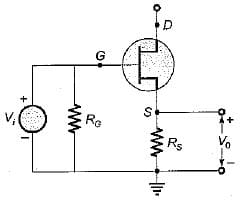Solution:

The small signal equivalent circuit of common-drain amplifier is shown below.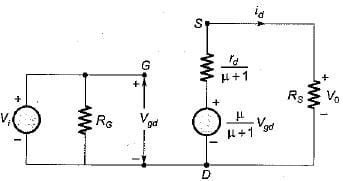Using Thevenin’s theorem, the output voltage is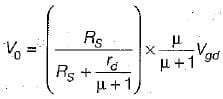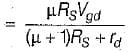(where, Vgd = Vi = input voltage)
∴ Voltage gain is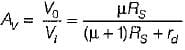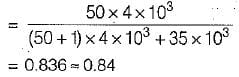QUESTION: 2

### The value of V0/Vin for the circuit shown below is (Assume M1 in saturation)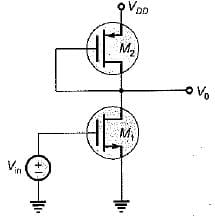Solution:

M2 is also saturated because its gate and drain are shorted together. The small signal circuit is shown below: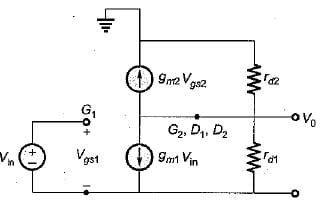Here,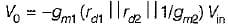For most MOSFET transistor, rd1 and d2 are much greater than 1/gm2.
Hence,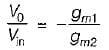Now,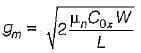∴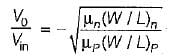= Required voltage gain

QUESTION: 3

### Consider the following statements: 1. In most linear applications of field-effect transistors the device is operated in the. constant-current portion of its output characteristics. 2. In the region before pinch-off, where VDS is small, the FET is useful as a voltage variable resistors (VVR). 3. The VVR can be used in AGC amplifier to vary the voltage gain of multistage amplifier. Q. Which of the above statements is/are correct?

Solution:
QUESTION: 4

In the saturation region, the I- VGS characteristics of a MOSFET are

Solution:

For amplifier operation, MOSFET is always operated in saturation region.
Since,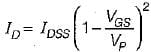in saturation region,therefore the ID - VGS characteristics of a MOSFET are quadratic as evident from the above equation.

QUESTION: 5

The source-bias circuit provides DC biasing for the FET. The resistance from gate to ground (RS) in that circuit is necessary because, without it,

Solution:
QUESTION: 6

In an FET common-source high frequency amplifier, which one of the following is the correct expression for input capacitance Ci ?

Solution:
QUESTION: 7

A JFET is set up as a source follower. Given, μ = 200, rd = 100 kΩ and source load resistance RL = 1 kΩ. The output resistance R0 is given approximately by

Solution:

We know that,
μ = gmrd
or,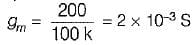Output resistance,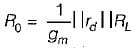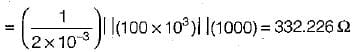QUESTION: 8

A FET tuned amplifier with gm = 5 mA/V, rd = 20 kΩ has a resonant impedance of 20 kΩ. The gain at resonance is given by

Solution:

AV = Gain at resonance = gmR
Here, R = rd ║Rmax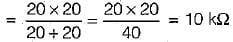∴ AV = 5x 10-3 x 10 x 103 = 50

QUESTION: 9

The unity gain bandwidth fT of a JFET is given by

Solution:
QUESTION: 10

The transconductance for a JFET having  IDSS = 8 mA, VP = -5 V and biased to operate at VGS = - 1.8 volt will be given by

Solution: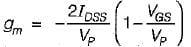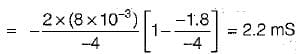Use Code STAYHOME200 and get INR 200 additional OFF Use Coupon Code